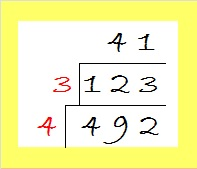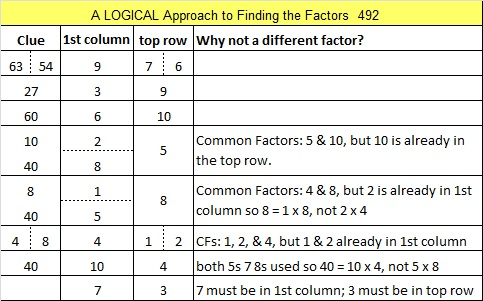# Simplifying √492 and Level 5

492 is the sum of some consecutive prime numbers (actually two different ways: see the comments). I’ll list those primes in the comments in about a week unless somebody else beats me to it. (abyssbrain beat me to it.)

Simplifying the square root of 492 is as easy as 1-2-3:

92 can be evenly divided by 4, so 492 is also divisible by 4. If I wanted to find √492, I would make a little cake and divide 492 by 4.123 is not divisible by 4 or by 9, but it is divisible by 3 so I will do that division next and get a quotient of 41, a prime number. Now I will take the square root of everything on the outside of the cake and multiply them together: √492 = (√4)(√3)√(41) = (√4)(√123) =2√123Print the puzzles or type the solution on this excel file: 10 Factors 2015-05-11

—————————————————————————————————

• 492 is a composite number.
• Prime factorization: 492 = 2 x 2 x 3 x 41, which can be written 492 = (2^2) x 3 x 41
• The exponents in the prime factorization are 2, 1, and 1. Adding one to each and multiplying we get (2 + 1)(1 + 1)(1 + 1) = 3 x 2 x 2 = 12. Therefore 492 has exactly 12 factors.
• Factors of 492: 1, 2, 3, 4, 6, 12, 41, 82, 123, 164, 246, 492
• Factor pairs: 492 = 1 x 492, 2 x 246, 3 x 164, 4 x 123, 6 x 82, or 12 x 41
• Taking the factor pair with the largest square number factor, we get √492 = (√4)(√123) = 2√123 ≈ 22.181073—————————————————————————————————## 2 thoughts on “Simplifying √492 and Level 5”

1.abyssbrain

Ha! I found two solutions 🙂 First, I quickly found that it’s the sum of two consecutive primes.

241 + 251 = 492

But I knew that it’s not that easy, so I tried to find another solution, and here it is,

492 = 71 + 73 + 79 + 83 + 89 + 97

•ivasallay

Oops, disorganized me forgot about 241 + 251. Thanks for finding and posting both solutions!

This site uses Akismet to reduce spam. Learn how your comment data is processed.